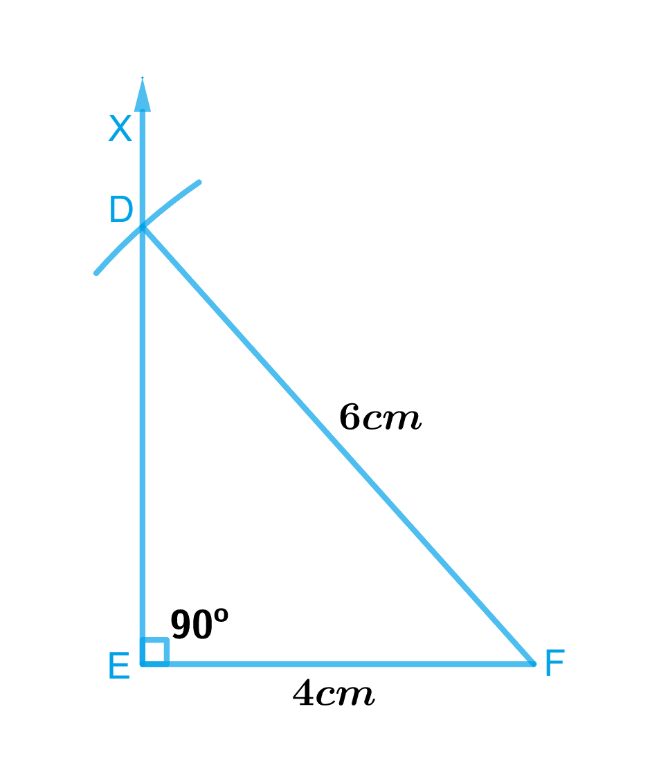# Ex.10.5 Q2 Practical Geometry Solution- NCERT Maths Class 7

Go back to  'Ex.10.5'

## Question

Construct a right-angled triangle whose hypotenuse is $$6\,\rm{ cm}$$ long and one of the legs is $$4\,\rm{ cm}$$ long.

Video Solution
Practical Geometry
Ex 10.5 | Question 2

## Text Solution

What is known?

Hypotenuse of a right-angled triangle and one of the legs.

To construct:

A right-angled triangle whose hypotenuse is $$6\,\rm{ cm}$$ long and one of the legs is $$4\,\rm{ cm}$$ long.

Reasoning:

To construct a right-angled triangle whose hypotenuse is $$6\,\rm{ cm}$$ long and one of the legs is $$4 \,\rm{cm}$$ long, draw a rough sketch and mark the measures. Remember to mark the right angle
and follow the steps given below.

Steps:Steps of construction

1. Draw a line segment $$EF$$ of length $$4\,\rm{ cm}.$$
2. At $$E$$, draw $$EX\,\text{perpendicular}\, EF.$$
3. With $$F$$ as centre, draw an arc of radius 6cm which should intersect $$EX$$ at point $$D.$$
4. Join $$D$$ with $$F$$.

$$ΔDEF$$ is the required triangle.

Learn from the best math teachers and top your exams

• Live one on one classroom and doubt clearing
• Practice worksheets in and after class for conceptual clarity
• Personalized curriculum to keep up with school